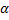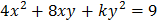# Consider the triangle formed by the lines Column-I Column- II (A) Values ofiflies inside triangle (p)(B) Values ofiflies inside triangle (q)(C) Values ofiflies inside triangle (r) No value of(D) Value ofiflies outside triangle   (s)CODES :   A B C D     a) P q r s     b) s r q p     c) q r s t     d) p q t s

## Question ID - 54548 :- Consider the triangle formed by the lines Column-I Column- II (A) Values ofiflies inside triangle (p)(B) Values ofiflies inside triangle (q)(C) Values ofiflies inside triangle (r) No value of(D) Value ofiflies outside triangle   (s)CODES :   A B C D     a) P q r s     b) s r q p     c) q r s t     d) p q t s

3537

(b)

1.2. Clearly, pointlies on the-axis, which is not intersecting any side of triangle, hence no suchexistsNext Question :
 Column-I Column- II (A) The valuefor whichis the equation of a pair of straight lines is (p) 3 (B) If the sum of the slopes of the lines given byis four times their product, then the value ofis (q)(C) If the gradient of one of the linesis twice that of the other, then(r) 2 (D) If the linesare equally inclined to the lines, then the value ofcan be (s) 4 CODES : A B C D a) S,r p,q p,q,r,s t b) p,q r,s t q c) p,q,r,s p s t d) s r p,q p,q,r,s